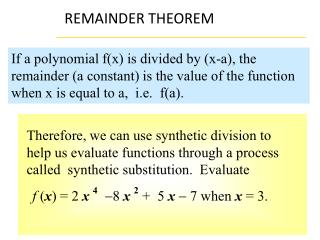DownloadDownload Presentationf ( x ) = 2 x 4 - 8 x 2 + 5 x - 7 when x = 3.

# f ( x ) = 2 x 4 - 8 x 2 + 5 x - 7 when x = 3.

Télécharger la présentation## f ( x ) = 2 x 4 - 8 x 2 + 5 x - 7 when x = 3.

- - - - - - - - - - - - - - - - - - - - - - - - - - - E N D - - - - - - - - - - - - - - - - - - - - - - - - - - -
##### Presentation Transcript

1. Therefore, we can use synthetic division to help us evaluate functions through a process called synthetic substitution. Evaluate f (x) = 2 x4-8 x2 + 5 x- 7 when x = 3. REMAINDER THEOREM If a polynomial f(x) is divided by (x-a), the remainder (a constant) is the value of the function when x is equal to a, i.e. f(a).

2. Polynomial in standard form 3 Coefficients x-value SOLUTION 2x4 + 0x3– 8x2 + 5x– 7 Polynomial in standard form 2 0 –8 5 –7 3• Coefficients 6 18 30 105 35 10 98 2 6 The value of f(3) is the last number you write, In the bottom right-hand corner. Here f(3)=98

3. Evaluate f(x) = 2x4-8x2 + 5x- 7 when x = 3. Using direct substitution to evaluate polynomial functions is another alternative, lets compare. f(x)=2x4-8x2+5x-7 Find f(3) f(3)=2(3)4-8(3)2+5(3)-7 f(3)= 2(81)-8(9)+15-7 f(3)=162-72+15-7 f(3)=98

4. Use synthetic substitution f(x) = 3x4-2 x3 + x2- 2 find f(4)

5. Polynomial in standard form 4 Coefficients x-value SOLUTION 3x4 – 2x3 + x2 + 0x– 2 Polynomial in standard form 3 -2 1 0 –2 4• Coefficients 12 40 164 656 164 41 654 3 10 The value of f(4) is the last number you write, In the bottom right-hand corner. Here f(4)=654

6. Use synthetic substitution SOLUTION • f(3)=0, what does that mean? • Two very important concepts. • 3 is a zero of the function. • x-3 is a factor of the polynomial.

7. If P(a)=0, then x-a is a factor of P(x). Conversely, if x-a is a factor of P(x), then P(a)=0 Factor Theorem

8. SOLUTION

9. RATIONAL ZERO THEOREM If a polynomial function has integer coefficients, then every rational zero of P(x) has the form where p are the factors of the constant and q are the factors of the leading coefficient

10. RATIONAL ZERO THEOREM Use the rational zero theorem to list the POSSIBLE rational zeros. p=1, 2, 3, 4, 6, 12 Identify p and q q=1, 2 Simplify and eliminate duplicates.

11. HOMEWORK Pages 379-381; 1-23 ODD